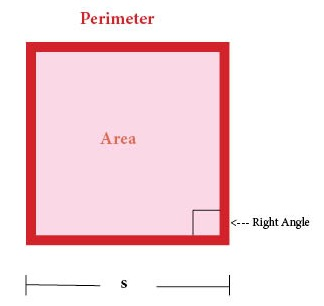# Parallelogram

Parallelogram = Flat Four-Sided Shape in which Opposite Sides are Parallel

# Cube

Cube = Three-Dimensional Shape with Six Square Faces (with all right angles)# Square

Square = Flat Shape with Four Equal Sides (with four right angles)# SquaringNumber Squared = Number × Itself = Number²